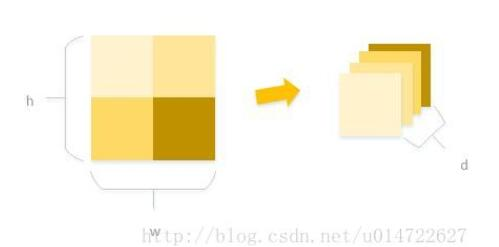# 在python2.7中用numpy.reshape 对图像进行切割的方法

更新时间：2018年12月05日 11:09:19   作者：jdefla我要评论numpy.reshape 的文档：点击打开链接

```import cv2
import numpy as np

im=cv2.imread('animal007.jpg',cv2.IMREAD_GRAYSCALE)
h= 300
w= 300
patchsize=100
d=h*w/patchsize/patchsize
print d

#crop the image to h x w
im=np.array(im[: h ,: w ])

#this gets wrong answer
im_wrong1=im.reshape((patchsize,patchsize,-1))
im_wrong7= im.reshape((patchsize,patchsize,-1),order='F')
im_wrong2=im.reshape(( h ,patchsize,-1)).reshape((patchsize,patchsize,-1))
im_wrong3=im.reshape((patchsize, w ,-1)).reshape((patchsize,patchsize,-1))
im_wrong4= im.reshape(( h ,patchsize,-1),order='F').reshape((patchsize,patchsize,-1))
im_wrong5= im.reshape(( h ,patchsize,-1),order='F').reshape((patchsize,patchsize,-1),order='F')
im_wrong6= im.reshape((patchsize, w ,-1),order='F').reshape((patchsize,patchsize,-1),order='F')

#this only works for h=w, i dont know why
im2= im.reshape((patchsize, w ,-1),order='F').reshape((patchsize,patchsize,-1))
im2_0=im2[:,:,no]
cv2.imwrite('im2_0.jpg',im2_0)

no=4;
imw=im_wrong1[:,:,no]
cv2.imwrite('imw1_0.jpg',imw)
imw=im_wrong2[:,:,no]
cv2.imwrite('imw2_0.jpg',imw)
imw=im_wrong3[:,:,no]
cv2.imwrite('imw3_0.jpg',imw)
imw=im_wrong4[:,:,no]
cv2.imwrite('imw4_0.jpg',imw)
imw=im_wrong5[:,:,no]
cv2.imwrite('imw5_0.jpg',imw)
imw=im_wrong6[:,:,no]
cv2.imwrite('imw6_0.jpg',imw)
imw=im_wrong7[:,:,no]
cv2.imwrite('imw7_0.jpg',imw)

```